# Thermal sensation in transient conditions at subway stations during the winter

Thermal sensation in transient conditions at subway stations during the winter

Huijuan Zhou*Meijie Jia Baoxun Liu Zhi Chen

Beijing Key Lab of Urban Intelligent Traffic Control Technology, North China University of Technology, Beijing 100144, China

ENNEW Cloud Data Service Co., Ltd, Langfang, Hebei 065001, China

Corresponding Author Email:
zhouhuijuan@ncut.edu.cn
Page:
371-377
|
DOI:
https://doi.org/10.18280/ijht.350220
|
Accepted:
|
Published:
30 June 2017
| Citation

OPEN ACCESS

Abstract:

Due to the huge difference between outdoor and indoor temperatures during the winter, subway riders inevitably feel thermal discomfort entering and exiting the air-conditioned station interiors and trains. This paper attempts to identify the thermal sensation response resulted from the lagging effect of the transient thermal conditions in a subway station, which consist of “cold-neutral-warm” and “warm-neutral-cold” phases. The results show that the transient variation in ambient temperature causes abrupt sensation responses and affects thermal sensation vote (TSV). Based on multiple conditions and effective temperature difference, the author examines the temporal TSV variations in both the hot transition and cold transition, and calculates the exact values by the respective equations. Moreover, the thermal sensation expectation is used to represent the thermal sensation of unidirectional or bidirectional passenger flows moving across different sections in the transitional space, and is calculated based on the TSV value in unidirectional or bidirectional passenger flows. The findings shed new light on the optimization of subway operation mode.

Keywords:

thermal sensation, passenger comfort, effective temperature, transitional space, subway station

1. Introduction

During the winter, there is a huge difference between outdoor and indoor temperatures. Subway riders entering and exiting the station inevitably experience the variation in thermal sensation. Specifically, the passengers moving in the direction from the outdoor, the hall, the corridor, the platform to the train go through the “cold-neutral-warm-hot” conditions, while those moving in the opposite direction go through the “hot-warm-neutral-cold” conditions. The space between the hall and the platform is known as the transitional space. Subway riders usually pass through two spatial transitions: from the outdoor to the transitional space, and from the transitional space to the train. With the changing ambient temperature, the spatial transitions bring changes to thermal sensation, induce discomfort, and thereby suppress the ridership.

The thermal sensation and comfort of passengers depend on the thermal environment of the subway station, which is, in turn, determined by the air temperature fluctuation and vertical temperature distribution of the station . Probing into the thermal environment of subway stations, most scholars focused on the platform and the measurement of actual temperatures [2-5]. For instance, Li et al.  evaluated the thermal environment of an air-conditioned station by the computational fluid dynamics method. Marzouk  monitored thermal comfort in subways through building information modeling. Han  examined the indoor environment of subway stations and the thermal comfort of passengers during three seasons in Seoul.

The transient conditions in indoor spaces, including the previously defined “transitional spaces”, are by dynamicity, variability, instability and fluctuations [9-11]. The transient conditions are made complicated by various variables, such as temperature difference, solar radiation, wind, and local microclimates. Better thermal comfort in transitional spaces is of great significance because it promotes business activities and enhances the quality of life .

Much research has been done on thermal sensation. In 1967, Gagge  investigated the thermal comfort in two different thermal environments; simulating the physiological parameters of several objects, he concluded that thermal sensation either stay ahead or lag behind the temperature variation. Through the analysis of the thermal sensation statistics on some objects, Fanger  established an expression of thermal sensation and environmental parameters, and visualized thermal sensation by presenting the Predicted Mean Vote (PMV) and Predicted Percentage of Dissatisfied (PPD). Woyn  argued that thermal sensation depended on the variation in ambient temperature, especially the sudden changes to the thermal environment. Chen  explored thermal sensation and skin temperature, and compared thermal sensations between the two genders. Kuno  highlighted that the physiological adaptation was a gradual process, even if the movement was only from one room to the other or from the indoor to the outdoor. In 2011, Ma Qian  analyzed three different transitional spaces and proved that the temperature and wind speed were the main influence factors of thermal sensation. Ding Qianru  dug into the duration and variation of the leading or lagging thermal sensations. The above studies demonstrate that the ambient temperature exerts physiological and psychological impacts to thermal sensation.

Taking a subway station of Beijing as an example, this paper tracks the changing thermal sensation in winter, summarizes the adaptive behaviors of respondents in transient thermal conditions, and identifies the relationship between thermal effect and sensation response. The purpose of this research is to disclose how the thermal transition of “cold-neutral-warm-hot” or “hot-warm-neutral-cold” influences the thermal sensation and acceptability of each rider, and thereby guides the design strategies and operation mode of the subway. The results help to clarify the relationship between the ambient temperature and the thermal comfort acceptability of the transitional space.

2. Methodology

2.1 Field test

In this research, the author selects a subway station of Beijing as the object and defines the study area as the entire space from the outdoor, the hall, the corridor, the platform to the train. The passengers’ movements are divided into two processes, each of which contains four phases. The entering process covers the phase from the outdoor to the hall (the O-H phase), the phase from the hall to the corridor (the H-C phase), the phase from the corridor to the platform (the C-P phase) and the phase from the platform to the train (the P-T phase); the exiting process is the exact opposite to the entering phase, consisting of the phase from the train to the platform (the T-P phase), the phase from the platform to the corridor (the P-C phase), the phase from the corridor to the hall (the C-H phase), and the phase from the hall to the outdoor (the H-O phase). The instruments used in this study include a temperature and humidity meter and an anemometer.

2.2 Questionnaire survey

2.2.1 Respondents and questions

Table 1. Statistical information of participants

 Statistical information Male Female Age range/year of age 22~25 21~25 Average age/year of age 23 23 Height range/cm 168~176 152~164 Average height/cm 172 158 Weight range/kg 54~66 45~55 Average weight/kg 63 49

In this survey, 20 respondents were selected from second-year graduate students at North China University of Technology (NCUT), including 20 males and 8 females. They were asked to enter the subway station in normal clothes during the winter and answer the questions on gender, age, height, weight, clothes, and thermal sensation. Table 1 shows the respondents’ physical information and Table 2 lists the voting standard of thermal comfort.

Table 2. Thermal sensation voting value

 Thermal sensation Thermal sensation vote cold -3 cool -2 Slight cool -1 neutral 0 slight warm 1 warm 2 hot 3

2.2.2 Tracking test

The tracking test is designed to trace the variation in thermal sensation when passengers go through different thermal environments. Previous studies put the appropriate adaptation phase at 5-20mins  [20-22]. Thus, the students were required to sit still for 20mins before casting the first vote. Prior to the O-H phase of the entering process, they stayed outside the station for 20mins to eliminate the effect of the previous thermal environment. After 20mins, they finished answering the first questionnaire and entered the hall. In the following 20mins (the H-C phase), the students answered one question every one minute in the first 10mins, and one question every two minutes in the second 10mins. Prior to the third phase (the C-P phase), they had to stay in the corridor for 20mins before entering the platform. The last phase (the P-T phase) is similar to the first three phases.

2.3 Field test and analysis of questionnaire results

## 1.png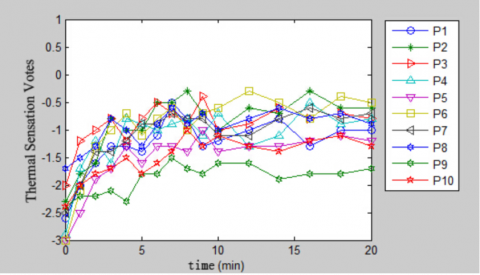Figure 1. Thermal sensation changes from outdoor to hall

## 2.png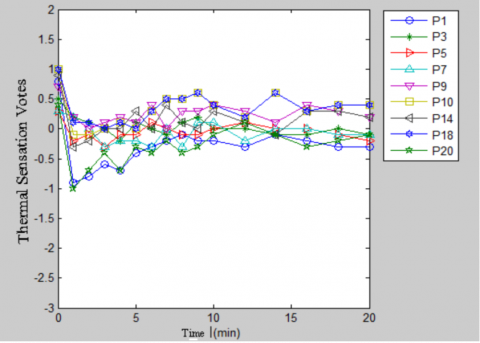Figure 2. Change of individual thermal sensation during cold transition

Out of the various data and curves, the results of 10 respondents are selected for the investigation. Figure 1 describes the thermal sensations in different thermal environments. As the respondents move from the outdoor to the hall, the air temperature changes from 8.8°C to 10.4°C, the relative humidity from 33.4% to 33.2% and the air velocity from 0.5m/s to 0.3m/s. As shown in the figure, the thermal sensation differs from respondent to respondent. The result may be subject to the influence of the difference in thermal sensitivity or clothes, but almost every vote value rises when the respondents enter a warmer thermal environment.

The reverse process (from a warm thermal environment to a cold thermal environment) is also tested in this research. Figure 2 depicts the variation in thermal sensation as the respondents move from (22.1°C, 33.4%, 0m/s) to (13.9°C, 33.2%, 0m/s). When the respondents enter a colder environment, the thermal sensations of some respondents reach the minimum, then slowly rebound, and eventually stabilize. This phenomenon is called the “leading cold effect” . The thermal sensations of the other respondents slowly decline and eventually reach a steady state.

In short, the two thermal transition processes manifest obvious individual differences in thermal sensation. The vote value of one respondent cannot reflect the entire environment. Hence, the author decides to evaluate the thermal sensation based on the average vote value of multiple respondents.

2.4 Data processing

2.4.1 Comfort temperature

With the changing of the seasons and the ambient temperature, people would take proper measures to keep the body comfortable, namely changing clothes, adjusting the temperature on the air-conditioner, and controlling the fan speed. According to De Dear’s  research, the relationship between the comfortable temperature and the outdoor temperature is expressed as: $T_{C}=17.8+0.31 T_{0}$

Where $T_{C}$ is the comfortable temperature and $T_{0}$ is the average monthly outdoor temperature. The value of $T_{0}$ is calculated from the exponentially weighted moving average.

$T_{r m}(1-\alpha)\left\{T_{t-1}+\alpha T_{t-2}+\alpha^{2} T_{t-3}+\ldots\right\}$

where $T_{r m}$  is the moving average temperature; $\alpha$ is a constant between 0 and 1; $T_{t}$ is the temperature at t in a time series. $T_{r m}$ and $T_{C}$ have the strongest correlation .

2.4.2 Effective temperature

The thermal sensation and comfort of the human body are the combined effect of multiple factors. For the sake of simplicity, temperature, relative humidity, air velocity and other environmental parameters are converted into the effective temperature ( $T_{E}$ ).

$T_{E}=37-\frac{37-T_{a}}{\left[0.68-0.0014 R H+\frac{1}{1.76+1.4 v^{0.75}}\right]}-0.29 T_{a}(1-\mathrm{RH} / 100)$        (1)

where $T_{a}$ is temperature (ᵒ s); RH is air humidity (%), v is air velocity (m/s).

3. Analysis of Thermal Sensation in Hot Transition, Cold Transition and Different Sections

Since the subway station is warmer than the outside in winter, it is inevitable for subway riders to go through different thermal environments. The temperature rises as they move from the outside to the hall, and drops as they leave the station. The transfer from a cool environment to a neutral or warm one is called the hot transition, and the transfer in the opposite direction is called the cold transition.

This section first analyzes the relationship between thermal sensation and effective temperature difference, and then discusses the temporal variation in thermal sensation in hot transition, cold transition and different sections.

3.1 The relationship between thermal sensation and effective temperature difference

The effective temperature difference ( $\Delta T_{E}$ ) refers to the difference between effective ambient temperature ( $T_{E}$ ) and comfort temperature ( $T_{c}$ ). When $\Delta T_{E}$ changes, the thermal sensation of each respondent will change accordingly. Taking the average of all the original thermal sensation votes, the author acquires the thermal sensation votes (TSVs) of $\Delta T_{E}$ under 28 conditions from -11.58ᵒC to 2.36ᵒC. The TSVs are illustrated in the scatterplot in Figure 3.

## 3.png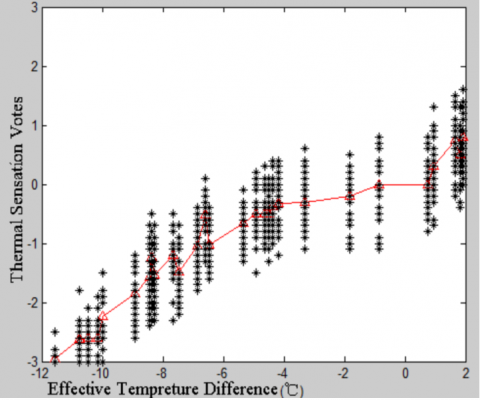Figure 3 Thermal sensation vote scatter plot

According to the figure, the value TSV decreases at an increasingly faster rate with the decline in $\Delta T_{E}$. This means the thermal sensation varies little with the effective temperature approximating the comfort temperature ( $\Delta T_{E} \in[-5,1.0]$ ), and plunges with the effective temperature deviating from the comfort temperature. When $\Delta T_{E}$ continues to fall, the thermal sensation votes will reach the minimum constant value of -3.

In light of the strong affinity between the effective temperature in this research and the skin temperature, it is meaningful to take for reference the close relationship between skin temperature and thermal sensation. According to Jin Quan et al. , the relationship is expressed as below.

$T S V_{i}=\left\{\begin{array}{cc}{+3} & {\Delta T_{i}>\ln 4 / W_{i}} \\ {\exp \left(W_{i} \Delta T_{i}\right)-1} & {0 \leq \Delta T_{i} \leq \ln 4 / W_{i}} \\ {1-\exp \left(C_{i} \Delta T_{i}\right)} & {\ln 4 / C_{i} \leq \Delta T_{i} \leq 0} \\ {-3} & {\Delta T_{i}<\ln 4 / C_{i}}\end{array}\right.$     (2)

where TSViis the partial thermal sensation vote; $\Delta T_{i}$ is the difference between local skin temperature and local skin comfort temperature; Wi and Ci are regression coefficients.

Thus, the relationship between the overall thermal sensation and effective temperature difference can be expressed with the following function.

T S V=\left\{\begin{aligned} a(1-\exp (b \Delta T e)) & \ln (a /(a+3)) / b<\Delta T e<2.0 \\-3 & \Delta T e \leq \ln (a /(a+3)) / b \end{aligned}\right.    (3)

Where a and b are regression coefficients.

The dynamic characteristics of the variation in thermal sensation with independent variable $\Delta T_{E}$ is analyzed by the regression equation. The best-fit curve appears at R2= 0.9415, when a and b are 0.4241 and 0.1833, respectively. The curve is displayed in Figure 4.

## 4.png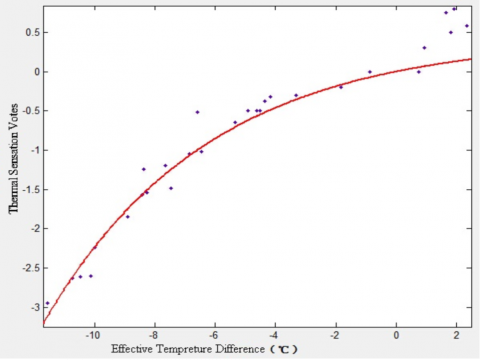Figure 4. Fitting curve of TSV and $\Delta T_{E}$

Thus, the relationship between the TSV and effective temperature difference is obtained as below.

$T S V=\left\{\begin{array}{rlrl}{0.4241\left(1-1 / \exp \left(0.1833 \Delta T_{E}\right)\right)} & {-11.39<\Delta T_{E}<2.36} & {} \\ {-3} & {\Delta T_{E}} { \leq-11.39}\end{array}\right.$      (4)

Whereas the experiment is performed in a cold environment, the function puts more emphasis on the cold environment than the warm environment.

3.2 Analysis of thermal sensation variation in hot transition

In winter, the process of entering the subway station is a typical hot transition. In the human body, the skin temperature variation is felt through cold and warm thermo-receptors. Under a sudden temperature change, these thermo-receptors would react strongly initially; the reaction intensity then declines rapidly and eventually reaches a stable state . Figure 5 shows the thermal sensation variation in hot transition under 9 conditions. As shown in this figure, when the respondents go through a hot transition, the initial thermal sensations change rapidly and sharply; then, the fluctuations are weakened and eventually stabilized. Figure 6 displays the absolute change rate of each thermal sensation vote in hot transition.

## 5.png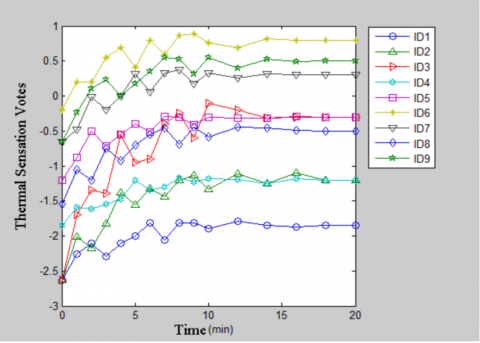Figure 5. TSV changes in hot transition

## 7.png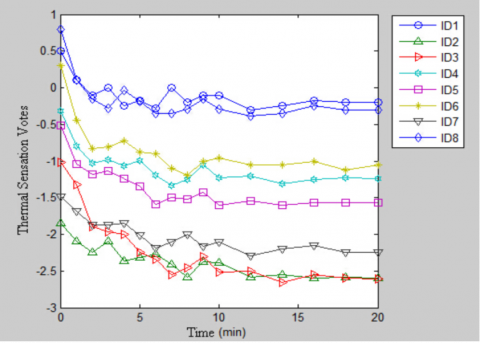Figure 6. TSV’s change rate

According to Figures 5 and 6, the TSV’s change rate hinges on the difference between the thermal sensations before and after the sudden temperature change. The larger the difference, the faster the change rate. The temporal variation in the TSV can be expressed as below:

$T S V_{u p}(t)=T S V_{1}-\left(T S V_{1}-T S V_{0}\right) / \exp (m t)$     (5)

where TVS0 and TVS1 are the TSVs before and after the sudden temperature change, respectively; $m$ is a constant; $t$is time.

Table 3. Regression analysis results under 9 conditions in hot transition

 No. Before transition After transition Separate regression m=0.3571 temperature (ᵒC) humidity (%) air speed (m/s) temperature (ᵒC) humidity (%) air speed (m/s) m R2 RMSE R2 RMSE ID1 8.8 33.4 0.5 10.4 33.2 0.3 0.369 0.845 0.092 0.845 0.092 ID2 7.1 33.4 0.5 8.8 33.4 0 0.336 0.920 0.126 0.918 0.127 ID3 8.8 33.4 0.5 13.9 33.2 0 0.354 0.919 0.197 0.919 0.198 ID4 7.1 33.2 0.3 10.4 33.4 0 0.307 0.908 0.063 0.895 0.067 ID5 7.1 33.4 0 13.9 33.2 0 0.393 0.902 0.081 0.897 0.083 ID6 13.9 33.2 0 22.1 33.4 0 0.375 0.883 0.105 0.882 0.105 ID7 10.4 33.3 0 20.2 33.4 0 0.356 0.887 0.104 0.887 0.104 ID8 10.4 33.2 0.3 13.5 33.4 0.1 0.350 0.891 0.105 0.891 0.106 ID9 10.4 33.3 0 21.6 33.5 0 0.375 0.896 0.108 0.894 0.109

Table 3 shows the regression results of the 9 conditions. Based on the regressed result of each condition, average all m values and replace the value m with the average value. Although the replacement will weaken the fitting effect, it does not harm the capacity of Equation (5) to formulate the temporal variation in the TSV. In the equation, m=0.3571 is the average value. Thus, we have:

$T S V_{u p}(t)=T S V_{1}-\left(T S V_{1}-T S V_{0}\right) / \exp (0.3571 t)$      (6)

3.3 Analysis of thermal sensation variation in cold transition

## 7.pngFigure 7. TSV changes in cold transition

Figure 7 presents the temporal variation in the TSV in cold transition under 8 different conditions. No “leading effect” occurs to the thermal sensation. The higher the TSV value prior to the transition, the faster the value changes after transition, and the greater the “leading effect”. With the TSV value decreases, the “leading effect” dwindles, the “lagging effect” becomes more prominent, and the TSV change rate gradually drops.

The temporal variation in the TSV in cold transition is expressed by the following function.

$T S V_{d o w n}(t)=T S V_{1}-\left(T S V_{1}-T S V_{0}\right) / \exp (q t)$     (7)

where TVS0 and TVS1 are the TSV values before and after the sudden temperature change, respectively; $q$ is a constant; $t$ is time.

Based on the previous analysis, the change rate is dependent on the TSV value prior to the transitions. Thus, Equation (7) can be rewritten as:

$\left\{\begin{array}{c}{T S V_{d o w n}(t)=T S V_{1}-\left(T S V_{1}-T S V_{0}\right) / \exp (m t)} \\ {q=a \cdot \exp \left(b\left(T S V_{0}+c\right)\right)}\end{array}\right.$    (8)

where the parameters are obtained through the regression analysis based on test data. The regression results are shown in Table 4. The three parameters are averaged as: a=0.8409, b=0.8272, and c=0.2804. The curve fitting effect with the average of the three parameters is no better than that of separate regressions, but the overall fitting effect is desirable. Therefore, Equation (8) is transformed as:

$\left\{\begin{array}{c}{T S V_{d o w n}(t)=T S V_{1}-\left(T S V_{1}-T S V_{0}\right) / \exp (q t)} \\ {q=0.840 \mathrm{exp}\left(0.8272\left(\mathrm{TSV}_{0}+0.2804\right)\right)}\end{array}\right.$    (9)

Table 4. Regression analysis results under 8 conditions in cold transition

 No. Before transition After transition separate regression a,b,c Temperature ᵒC Humidity % air speed m/s temperature ᵒC humidity% air speed m/s a b c R2 RMSE R2 RMSE D1 22.3 33.3 0 18.2 33.1 0.2 0.678 0.462 -0.184 0.788 0.084 0.698 0.100 ID2 10.4 33.2 0.3 10.2 33.3 0.6 2.597 2.010 0.587 0.826 0.086 0.815 0.088 ID3 12.8 33.5 0.3 11.3 33.3 1.0 1.017 1.078 -0.141 0.959 0.090 0.848 0.174 ID4 13.9 33.2 0.1 7.8 33.4 0.1 0.696 0.407 -0.368 0.859 0.088 0.804 0.104 ID5 12.4 33.4 0.3 10.7 33.4 0.5 0.364 0.829 0.616 0.911 0.081 0.787 0.126 ID6 20.2 33.4 0 12.2 33.3 0.3 0.496 0.424 0.379 0.906 0.103 0.804 0.148 ID7 11.2 33.2 0.1 9.5 33.2 1.2 0.464 0.841 0.704 0.885 0.071 0.839 0.084 ID8 22.1 33.4 0 13.9 33.2 0 0.413 0.567 0.650 0.900 0.085 0.812 0.117

3.4 Analysis of thermal sensation variation in each section of the subway station

In the subway station, the space from the hall to the platform is defined as the transitional space. The transitional space is further divided into a number of sections, denoted as A, B, C... In each section, the thermal environment is assumed to be constant, and the thermal sensation remains unchanged. According to the passenger flow direction, these sections fall into two categories: unidirectional passenger flow sections (Subgraphs 8(a) and (b)) and bidirectional passenger flow sections (Subgraph 8(c)). In unidirectional passenger flow sections, the passengers flow moves in one direction only, involving inbound or outbound passengers; in the bidirectional passenger flow sections, the passenger flow moves in two opposite directions, involving both inbound and outbound passengers.

The different flow directions between sections result in different thermal transition rates. In Subgraph 8(a), the passenger flow moves from section C to section B, and the thermal environment transition is denoted as HC-HB; in Subgraph 8(b), the passenger flow moves from section A to section B, and the thermal environment transition is denoted as HA-HB; in Subgraph 8(c), one part of the passenger flow undergoes the condition in 8(a), and the other part undergoes the condition in 8(b).

## 8.png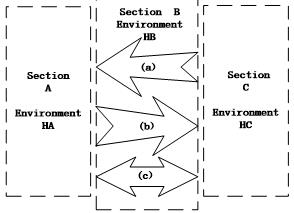Figure 8. Segmentation schematic for one-way and two-way passenger flow

From the above analysis, 20mins are needed to keep thermal sensation stable. The time spent travelling across one of the sections is not enough to stabilize the thermal sensation. Therefore, the passengers’ thermal sensation always changes and cannot reach stability. The concept of thermal sensation expectation is introduced for more accurate description of the thermal sensation of passengers moving between sections.

The thermal sensation expectation in the two categories of station sections should be calculated by the different methods below.

(1) Estimation of thermal sensation expectation in unidirectional passenger flow sections

For unidirectional passenger flow sections, the passengers either move from section A to section B or from section C to section B. In view of the temporal variation pattern of TSV mentioned above, the thermal sensation expectation is calculated based on the TSV value after transition:

1) If the TSV value increases, the case is a hot transition. According to Equation (5), the thermal sensation expectation is calculated as below.

$T S V_{E}\left(t_{0}\right)=T S V_{1}-\int_{0}^{t_{0}} \frac{T S V_{1}-T S V_{0}}{e^{a t}} t d t =T S V_{1}-\left(T S V_{1}-T S V_{0}\right) \int_{0}^{t_{0}} t e^{-a t} d t =T S V_{1}+\left(T S V_{1}-T S V_{0}\right)\left(t_{0} \exp \left(-a t_{0}\right)+\exp \left(-a t_{0}\right) / a-1 / a\right) / a$    (10)

where $\alpha=0.3571$; $t_{0}$ is transition time and $t_{0} \in(0,20)$ (min).

2) If the TSV value decreases, the case is a cold transition. According to Equation (9), the thermal sensation expectation is calculated as below.

$T S V_{E}\left(t_{0}\right)=T S V_{1}-\int_{0}^{t_{0}} \frac{T S V_{1}-T S V_{0}}{e^{a t}} t d t =T S V_{1}-\left(T S V_{1}-T S V_{0}\right) \int_{0}^{t_{0}} t e^{-a t} d t =T S V_{1}+\left(T S V_{1}-T S V_{0}\right)\left(t_{0} \exp \left(-a t_{0}\right)+\exp \left(-a t_{0}\right) / a-1 / a\right) / a$(11)

where $\alpha=0.8409 \exp \left(0.8272 \mathrm{SV}_{0}+0.2804\right)$ , $t_{0}$ is transition time and $t_{0} \in(0,20)$ (min).

3) If the TSV value remains unchanged, it means the TSV value does not change when passengers move between two adjacent sections despite the different thermal environments in these sections. The thermal sensation expectation of the next section is equal to that of the previous section, that is:

$T S V_{E}\left(t_{0}\right)=T S V_{1}$       (12)

(2) Estimation of thermal sensation expectation in bidirectional passenger flow sections

For bidirectional passenger flow sections, the passengers both move from section A to section B and from section C to section B. The TSV value should be the combination of the two values obtained in the preceding section. The proportion coefficient of passenger flow is introduced to represent the distribution of passengers in the two directions. Equations (10) and (11) are combined to calculate the thermal sensation expectation:

$\left\{\begin{array}{c}{T S V_{E}=\alpha T S V_{E, p 1}+(1-\alpha) T S V_{E, p 2}} \\ {\alpha=\frac{f l o w_{p 1} \overline{t}_{p 1}}{f l o w_{p 1} \overline{t}_{p 1}+f l o w_{p 2} \overline{t}_{p 2}}}\end{array}\right.$      (13)

where TSVE,p1 is the thermal sensation expectation for direction p1; TSVE,p2 is the thermal sensation expectation for direction p2; $a$ is the proportion coefficient of passenger flow; flowp1 is the passenger flow in direction p1; flowp2 is the passenger flow in direction p2; $\overline{t_{p 1}}$ is the average residence time of direction p1; $\overline{t_{p 2}}$ is the average residence time of direction p2.

4. Conclusion

During the winter, the passengers entering or exiting the transitional space in the subway station are subjected to sudden thermal sensation changes as the ambient temperature ranges between 7 and 22ᵒC. Through the field test, questionnaire survey and tracking test, this paper digs deep into the thermal sensation variation during the movement in the transitional space. The main findings are listed below.

(1) Thermal sensation and thermal comfort are sensitive to such environment parameters as temperature, relative humidity and air velocity. The effective temperature, on behalf of these environment parameters, has an effect on comfort temperature.

(2) The TSV changes with the effective temperature difference. Two regression equations are established for the TSV and effective temperature difference, respectively.

(3) Whether it is hot transition or cold transition, the thermal sensation changes rapidly under sudden temperature variations and eventually reaches a steady state. There is a “leading effect” in the cold transition. The model of temporal variation in TSV is built through the regression analysis of various actual conditions.

(4) The thermal sensation expectation is used to represent the thermal sensation of passengers moving across different sections in the transitional space, and is calculated based on the TSV value in unidirectional or bidirectional passenger flows.

This research mainly focuses on the TSV variation in the transitional space of a subway station in winter. The author only considers the cold ambient temperature and heavy clothes in winter and does not take account of the hot temperature and cool clothes in summer. In future, more attention should be paid to building a proper thermal transitional space based on energy conservation, human physiology, sensation, perception, and behavior.

Acknowledgment

The authors would like to acknowledge the support of National Key R&D Program of China (2016YFB1200402).

References

 Han J., Kwon S., Chun C. (2016). Chun indoor environment and passengers' comfort in subway stations in Seoul, Building and Environment, Vol. 104, pp. 221-231. DOI: 10.1016/j.buildenv.2016.05.008

 Ampofo F., Maidment G., Missenden J. (2004). Missenden, underground railway environment in the UK part 2: investigation of heat load, Appl. Therm. Eng., Vol. 24, No. 5, pp. 633-645. DOI: 10.1016/j.applthermaleng.2003.10.018

 Ampofo F., Maidment G., Missenden J. (2004). Missenden, underground railway environment in the UK: part 3: methods of delivering cooling, Appl. Therm. Eng., Vol. 24, No. 5, pp.647-659. DOI: 10.1016/j.applthermaleng.2003.10.019

 Choi Y.B. (2001). The evaluation of thermal environment and the planning of air conditioning and ventilation in the concourse of the station, Mag. Soc. Air-Conditioning Refrig. Eng., Vol. 30, No. 4, pp. 46-51.

 Kim H.R., Kim D.G., Kum J.S., Chung Y.H., Kim J.R., Kim M.S. (2009). The study for thermal environment improvement at subway station platform (Part 2: thermal environment according to supply outdoor temperature), Soc. Air-Conditioning Refrig. Eng. Korea, Vol. 6, pp. 561-564.

 Li Q., Yoshino H., Mochida A. (2009). CFD study of the thermal environment in an air-conditioned train station building, Build and Environment, Vol. 44, No. 7, pp. 1452-1465.  DOI: 10.1016/j.buildenv.2008.08.010

 Marzouk M., Abdelaty A. (2014) Monitoring thermal comfort in subways using building information modeling, Energy Buildings, Vol.  84, pp. 252-257. DOI: 10.1016/j.enbuild.2014.08.006

 Han J., Kwon S., Chun C. (2016). Indoor environment and passengers' comfort in subway stations in Seoul, Building and Environment, Vol. 104, pp. 221-231. DOI: 10.1016/j.buildenv.2016.05.008

 ZVold A. (2000). Thermal comfort at transient conditions, Proceedings of Passive Low Energy Architecture, pp.587-592.

 Hayashi T., Shibayama A., Hasebe R., Suzuki T., Horikawa S., Tanabe S., Kimura K. (1996). Field study on thermal comfort in transient spaces from outdoor to indoor, Indoor Air '96, Proceedings of the Seventh International Conference on Indoor Air Quality and Climate, Vol. 1, pp. 293-299.

 Hensen J.L.M. (1990). Literature review on thermal comfort in transient conditions, Building and Environment, Vol. 25, pp. 309-316. DOI: 10.1016/0360-1323(90)90004-B

 Raja I.A., Virk G.S. (2001). Thermal comfort in urban open spaces: a review, Proceedings of Moving Thermal Comfort Standards into the 21st Century, pp. 342-352.

 Gagge A.P., Stolwijk J.A.J., Saltin B. (1969). Comfort and thermal sensations and associate hysiological responses during exercise at various ambient temperatures, Environmental Research, Vol. 2, pp. 209-229. DOI: 10.1016/0013-9351(67)90002-3

 Fanger P.O. (1970). Thermal Comfort, Copenhagen: Danish Technical Press.

 Wyon D., Bruun N., Olesen S., Kjerulf-Jensen P., Fanger P. (1971). Factors affecting the subjective tolerance of ambient temperature swings, The 5th International, Congress for HVAC, Copenhagen, No. 1, pp. 87-107.

 Chen C.P., Hwang R.L., Chang S.Y., Lu Y.T. (2011). Effects of temperature steps on human skin physiology and thermal sensation response, Building and Environment, Vol. 46, No. 11, pp. 2387-2397. DOI: 10.1016/j.buildenv.2011.05.021

 Kuno S. (2007). A new concept of air-conditioning systems based on the theory of thermal comfort in transitional conditions, Proceeding of International Symposium on EcoTopia Science, pp. 1171-1174.

 Ma Q. (2011). Study on thermal comfort of campus buildings, Nanning: Guangxi University.

 Ding Q.R., Jin Q., Li X.L., Lin D.M. (2010). Effect of local thermal sensation on whole-body thermal sensation under stable non-uniform environment, Journal of Southeast University (English Edition), Vol. 26, No. 2, pp.238-242.

 Arens E., Zhang H., Huizenga C. (2006). Partial and whole-body thermal sensation and comfort- part ‖: non-uniform environmental conditions, Journal of Thermal Biology, Vol. 31, No. 1-2, pp. 60-66. DOI: 10.1016/j.jtherbio.2005.11.028

 Goto T., Toftum J., De D.R., Fanger P.O. (2006). Thermal sensation and thermo physiological responses to metabolic step-changes, International Journal of Biometeorology, Vol. 50, No. 5, pp.323-332. DOI: 10.1007/s00484-005-0016-5

 Zhang Y., Zhao R. (2009). Relationship between thermal sensation and comfort in non-uniform and dynamic environments, Building and Environment, Vol.  44, No. 7, pp. 1386-1391. DOI: 10.1016/j.buildenv.2008.04.006

 Dear R.J.D., Ring J.W., Fanger P.O. (1993). Thermal sensations resulting from sudden ambient temperature changes, Indoor Air, Vol. 3, No. 3, pp. 181-192. DOI: 10.1111/j.1600-0668.1993.t01-1-00004.x

 Dear R.J.D., Brager G.S. (2002). Thermal comfort in naturally ventilated buildings: revisions to ASHRAE standard 55, Energy and Buildings, Vol. 34, No. 6, pp. 549-561. DOI: 10.1016/S0378-7788(02)00005-1

 Humphreys M.A. (1974). Clothing and comfort of secondary school children in summertime, Electronic Imaging.

 Jin Q., Li X., Lin D., Shu H., Sun Y. (2012). Predictive model of local and overall thermal sensations for non-uniform environments. Building and Environment, Vol. 51, No, 7, pp.330-344. DOI: 10.1016/j.buildenv.2011.12.005

 Hensel H. (1982). Thermal sensations and thermo receptors in man, American Lecture.